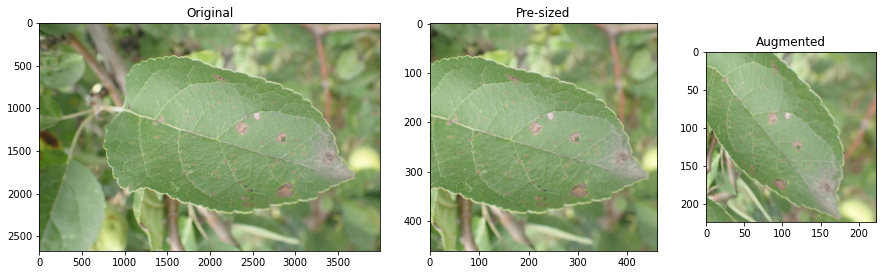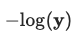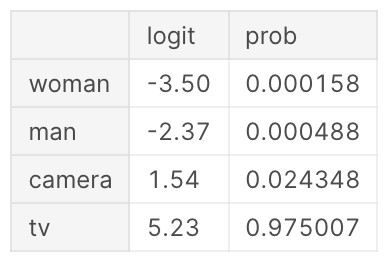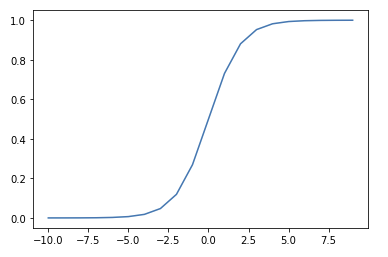• ## CardinalityCardinality refers to the number of elements a set contains.

• ## Binary Cross-Entropy Loss

Binary Cross-Entropy (BCE), also known as log loss, is a loss function used in binary or multi-label machine learning training.

It's nearly identical to Negative …

• ## PresizingPre-sizing is a technique for preparing images for training a neural network.

In standard image pipelines, images are resized to a size suitable for our …

• ## Categorical Cross-Entropy Loss

Categorical Cross-Entropy Loss Function, also known as Softmax Loss, is a loss function used in multiclass classification model training. It applies the Softmax Activation Function …

• ## Negative Log-LikelihoodNegative log-likelihood is a loss function used in multi-class classification.

Calculated as $-log(\textbf{y})$, where $\mathbf{\text{y …}}$

• ## Softmax Activation FunctionThe Softmax function converts a vector of numbers into a vector of probabilities that sum to 1. It's applied to a model's outputs (or Logits …

• ## Sigmoid Activation FunctionThe Sigmoid function squeezes numbers into a probability-like range between 0 and 1.1 Used in Binary Classification model architectures to compute loss on discrete …

• ## Domain ShiftWhen production data diverges significantly from the training dataset

• ## Out-of-domain dataWhen data is provided to a model that is significantly different from what it was trained on, it's referred to as out-of-domain data.

Howard et …

• ## Mean Absolute Difference - L1 Loss

Mean Absolute Difference (MAE) is a function for assessing Regression predictions. It's also as L1 Loss because it takes the L1 Norm of the error …